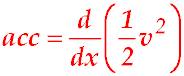Dr. J's Maths.com
Where the techniques of Maths
are explained in simple terms.

Calculus -Motion - Proof of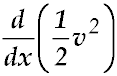.

In extension questions related to motion, we often need to express acceleration in terms of x (displacement). To prove this equation, follow the following steps:

 1. Write down the definition of acceleration:2. We need to introduce x so split the above definition as a chain rule statement.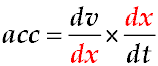3. Notice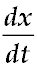is the definition of velocity v - so replace that derivative.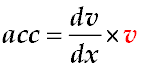4. To obtain v, we can differentiate with respect to v.5. Now cancel the "dv" terms.